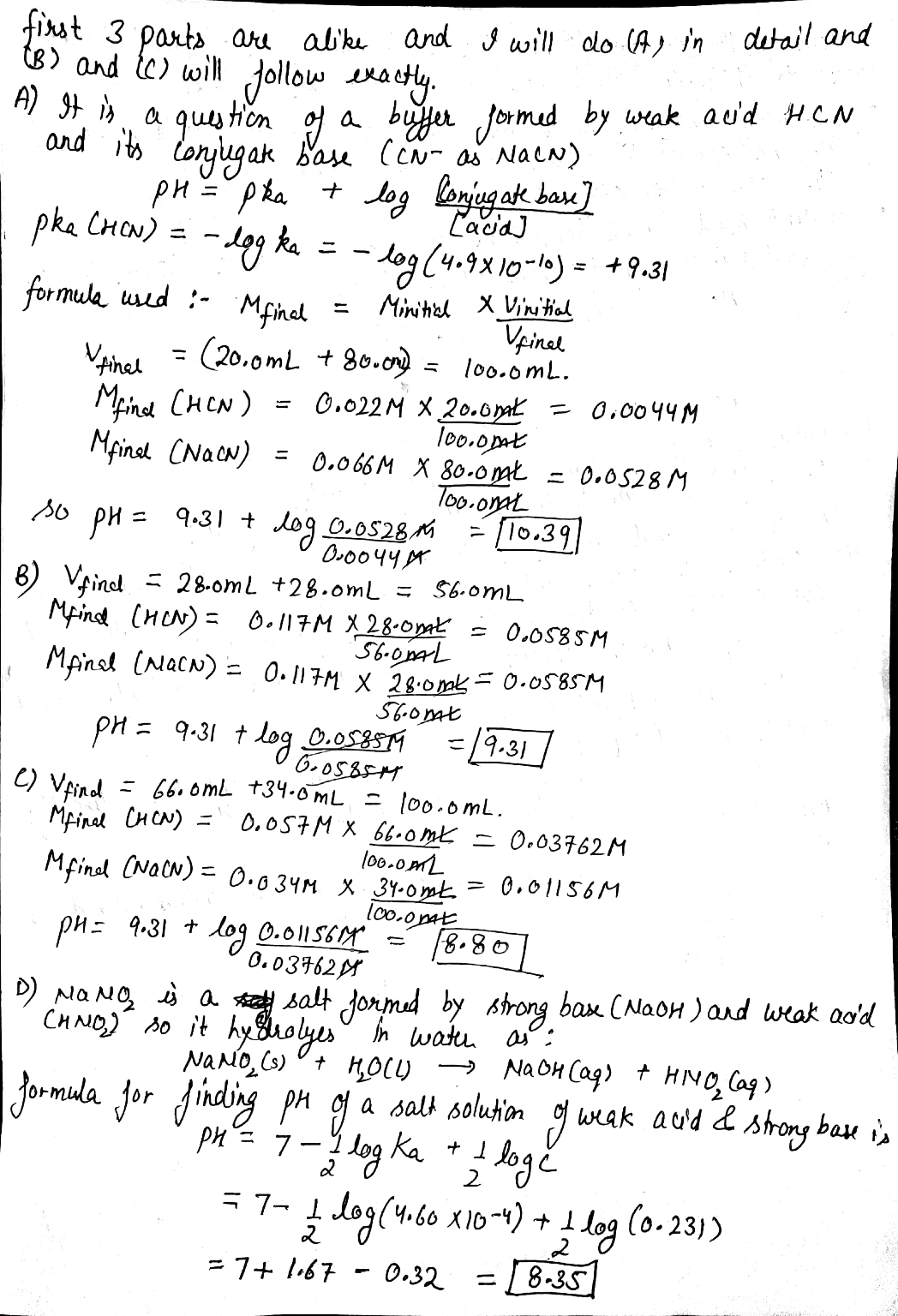In: Chemistry

# 78 A.) Calculate the pH of the solution that results from mixing 20.0 mL of 0.022...

78 A.) Calculate the pH of the solution that results from mixing 20.0 mL of 0.022 M HCN(aq) with 80.0 mL of 0.066 M NaCN(aq).

pH=

b. Calculate the pH of the solution that results from mixing 28.0 mL of 0.117 M HCN(aq) with 28.0 mL of 0.117 M NaCN(aq).

C. Calculate the pH of the solution that results from mixing 66.0 mL of 0.057 MHCN(aq) with 34.0 mL of 0.034 M NaCN(aq).. The Ka value for HCN is 4.9×10^(−10)

D.

Calculate the pH of a 0.231 M solution of NaNO2.. The ionization constant, ,Ka, for the acid, HNO2, is 4.60×10−4.

## Solutions

##### Expert Solution## Related Solutions

##### 1. Calculate the pH of a solution that results from mixing 15 mL of 0.13 M...
1. Calculate the pH of a solution that results from mixing 15 mL of 0.13 M HBrO(aq) with 11 mL of 0.1 M NaBrO(aq). The Ka value for HBrO is 2 x 10-9. 2. What is the buffer component ratio, (BrO-)/(HBrO) of a bromate buffer that has a pH of 7.91. Ka of HBrO is 2.3 x 10-9.
##### Calculate the pH of the buffer that results from mixing 60.0 ml of 0.250M HCHO2 and...
Calculate the pH of the buffer that results from mixing 60.0 ml of 0.250M HCHO2 and 15.0 ml of 0.500M NaCHO2?
##### Calculate the pH of the buffer that results from mixing 51.2 mL of a 0.400 M...
Calculate the pH of the buffer that results from mixing 51.2 mL of a 0.400 M solution of HCHO2 and 12.9 mL of a 0.697 M solution of NaCHO2. The Ka value for HCHO2 is 1.8 × 10−4 . Express your answer to two decimal places. (a) 4.15 (b) 2.38 (c) 3.39 (d) 12.8 (e) None of the above
##### What is the pH of the solution which results from mixing 35 mL of 0.50 M...
What is the pH of the solution which results from mixing 35 mL of 0.50 M NH3(aq) and 35 mL of 0.50 HCI(aq) at 25 degree C? (Kb for NH3 = 1.8*10^-5). Please show all work. The answer is pH=4.32
##### Calculate the pH of the solution that results from mixing 61.0mL of 0.052 MHCN(aq) with 39.0...
Calculate the pH of the solution that results from mixing 61.0mL of 0.052 MHCN(aq) with 39.0 mL of 0.025 M NaCN(aq).The ?a value for HCN is 4.9×10−10 pH= Calculate the pH of the solution that results from mixing 30.0 mL of 0.027 M HCN(aq) with 70.0 mL of 0.064 M NaCN(aq). pH= Calculate the pH of the solution that results from mixing 42.0 mL of 0.103 M HCN(aq) with 42.0 mL of 0.103 M NaCN(aq). pH=
##### Calculate the pH of the solution which results from mixing: 25.0mL of 0.100M HNO2 with 15.0mL...
Calculate the pH of the solution which results from mixing: 25.0mL of 0.100M HNO2 with 15.0mL of 0.100M NaOH
##### : Calculate the pH of a solution that results from 125 mL of 0.10M NH3 with...
: Calculate the pH of a solution that results from 125 mL of 0.10M NH3 with 250 mL of 0.10 M NH4Cl; Kb = 1.76 x 10-5 Calculate the pH when 0.010 mols of NaOH are added to 250.0 mL buffer containing 0.250 M acetic acid and 0.250 M sodium acetate. (pka = 4.74) A 50.0 mL sample of 0.200M NaOH is titrated with 0.200M nitric acid. Calculate the pH after 25.0 mL HNO3 has been added.
##### Calculate the pH of the solution that results from each of the following mixtures. 140.0 mL...
Calculate the pH of the solution that results from each of the following mixtures. 140.0 mL of 0.27 M  HF with 225.0 mL of 0.30 M  NaF 175.0 mL of 0.12 M C2H5NH2 with 265.0 mL of 0.20 M C2H5NH3Cl Express your answer using two decimal places.
##### Calculate the pH of a solution that results upon mixing 40ml of 0.09M NH3 with 8ml...
Calculate the pH of a solution that results upon mixing 40ml of 0.09M NH3 with 8ml of 0.18M HNO3
##### What is the pH of a solution that results from mixing together equal volumes of a...
What is the pH of a solution that results from mixing together equal volumes of a 0.160 M solution of acetic acid and a 0.080 M solution of sodium hydroxide?